# CBSE Solutions for Class 10 English

#### GSEB std 10 science solution for Gujarati check Subject Chapters Wise::

State quadratic equations have real roots or no real root :- −x2−2x−2=0

જવાબ : no real roots

State quadratic equations have real roots or no real root :-  x−1x−3=0, x≠0

જવાબ :  real roots

State quadratic equations have real roots or no real root :- 2x2−6x+3=0

જવાબ : real roots

State quadratic equations have real roots or no real root :- −13x2+3x+7=0

જવાબ : real roots

State quadratic equations have real roots or no real root :- 11x2−12x−1=0

જવાબ : real roots

State quadratic equations have real roots or no real root :- 9x2+x+3=0

જવાબ : no real roots

State quadratic equations have real roots or no real root :- 3x2+6x+1=0

જવાબ : real roots

State quadratic equations have real roots or no real root :- sx2+x+7=0

જવાબ : no real roots

Find the roots of the quadratic equation using square method :-2x2−7x+3=0

જવાબ : 2x2−7x+3=0 (x−7/4)2−(7/4)2+3/2=0
(x−7/4)2=49/16−3/2=0
(x−7/4)2=25/16
x−7/4=±5/4
or
x=1/2 or 3

Find the roots of the quadratic equation using square method :-  x2+4x−5=0

જવાબ : (x+4/2)2−(4/2)2−5=0
(x+2)2−9 = 0
(x+2)2= 9
x+2 = ±3
x=1 or -5

Find the roots of the quadratic equation using factorization technique :-

જવાબ : x2−11x+30=0
Solution- x2−11x+30=0
x(x-5)-(x-6)=0
Roots are 5 and 6

Find the roots of the quadratic equation using factorization technique :-

જવાબ : x2−3x−10=0
Solution- x2−3x−10=0
x(x−5)+2(x−5)=0
(x+2)(x−5)=0
So roots are x=-2 and 5

Find whether statement is True or False  :- Every quadratic equation will have rational roots

જવાબ : False

Find whether statement is True or False  :- if the roots of the quadratic equation are irrational, the coefficient of the term x will also be irrational

જવાબ : True

Find whether statement is True or False  :- if the roots of the quadratic equation are rational, the coefficient of the term x will also be rational.

જવાબ : True

Find whether statement is True or False  :- for k > 0,the quadratic equation 2x2+6x−k=02x2+6x−k=0 will definitely have real roots

જવાબ : True

Find whether statement is True or False  :- In a quadratic equation ax2+bx+c=0ax2+bx+c=0 ,if a and c are of opposite sign, then quadratic equation will definitely have real roots

જવાબ : True

In a quadratic equation ax2+bx+c=0ax2+bx+c=0 ,if a and c are of same sign and b is zero ,the quadratic equation has real roots

જવાબ : False

Find whether statement is True or False  :- A quadratic equation can have at most 2 real roots

જવાબ : True

Find whether statement is True or False  :- The roots of the equation x2−1=0x2−1=0 are 1,-1

જવાબ : True

Find whether statement is True or False  :- There are no reals roots of the quadratic equation x2+4x+5=0x2+4x+5=0

જવાબ : True

Find a natural number whose square diminished by 84 is thrice the 8 more of given number

જવાબ : 12

The roots of the quadratic equation x2+14x+40=0 are

જવાબ : (-4,-10)

State whether the equation is a quadratic equation or not a quadratic equation x5+x+20=0

જવાબ :
It is not a quadratic equation

The roots of the quadratic equation are real or non-real x2+2x+5=0

જવાબ : Non-real

Find the roots of 6x2−√2x−2=0 by the factorisation of the corresponding quadratic polynomial.

જવાબ : √23,√25−23,25

Find Whether the equation is a quadratic equation and isn’t? :- x3−x2+2x+1=(x+1)3

જવાબ : quadratic equation

Find Whether the equation is a quadratic equation and isn’t? :- (x+2)(x−1)=x2−2x−5

જવાબ : not quadratic equation

Find Whether the equation is a quadratic equation and isn’t? :- x2+3x=(−1)(1−3x)2

જવાબ : a quadratic equation

Find Whether the equation is a quadratic equation and isn’t? :- (x+2)2=2(x+3)

જવાબ : quadratic equation

State the quadratic equations have real roots, no real roots :-2x2−5x+3=0

જવાબ : y= 3/2 or 1

State the quadratic equations have real roots, no real roots :-3y2+4y=2(y+4)

જવાબ : y= -2 or 4/3

State the quadratic equations have real roots, no real roots :-14x+4x2=2x−5

જવાબ : y=-1 or 9/10

State the quadratic equations have real roots, no real roots :-9−y−10y2=0

જવાબ : y= -1 or 9/10

State the quadratic equations have real roots, no real roots :-11x2−31x−6=0

જવાબ : x= 3 or -2/11

State the quadratic equations have real roots, no real roots :-y2+(y+2)2=580

જવાબ : y=16 or -18

State the quadratic equations have real roots, no real roots :-x2+(7−x)2=25

જવાબ : x= 3 or 4

State the quadratic equations have real roots, no real roots :-2x2−x−2=0

જવાબ : Real roots

State the quadratic equations have real roots, no real roots :-2x2−6x+3=0

જવાબ : Real roots

State the quadratic equations have real roots, no real roots :-10x2+3x+7=0

જવાબ : No real roots

State the quadratic equations have real roots, no real roots :-11x2+5x+1=0

જવાબ : No real roots

State the quadratic equations have real roots, no real roots :-9x2+x−3=0

જવાબ : Real roots

State the quadratic equations have real roots, no real roots :-3x2+5x+1=0

જવાબ : Real roots

State the quadratic equations have real roots, no real roots :- x2+8x+7=0

જવાબ : Real roots

Find the roots with the quadratic equations :-  6x2 – 7x + 2 =0

જવાબ : 2/3 , ½

Find the roots with the quadratic equations :-  x2 – 43x +42 = 0

જવાબ : 1, 42

Find the roots with the quadratic equations :-  x2 - 5x -6 = 0

જવાબ : -1, 6

Find the roots with the quadratic equations :-  x2 + 3x +2=0

જવાબ : -1, -2

Find the roots with the quadratic equations :-  x2 + 6x +5=0

જવાબ : -5,-1

Had Ram scored 10 more marks in her mathematics test out of 30 marks, 9 times these marks would have been the square of her actual marks. How many marks did she get in the test?

જવાબ : 15

In the roots of the following quadratic equation: 23x2 – 5x + 3 = 0 (2011D)

જવાબ :We have, 2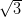x2 – 5x += 0
Here, a = 2, h = -5, c =D = b2 – 4ac
D = (-5)2 – 4 (2)()
= 25 – 24 = 1Solve for x: 4x2 – 4ax + (a2 – b2) = 0 (2011OD)

જવાબ : 4x2 – 4ax + (a2 – b2) = 0
[4x2 – 4ax + a2] – b2 = 0
[(2x)2 – 2(2x)(a) + (a)2] – b2 = 0
(2x – a)2 – (b)2 = 0
(2x – a + b) (2x – a – b) = 0
2x – a + b = 1 or 2x – a – b = 0
2x = a – b or 2x = a + b

x =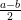or x =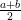Solve for x: 3x2 – 26x + 2 = 0 (2012D)

જવાબ : 3x2 – 2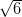x + 2 = 0
3x2 –x –x + 2 = 0x (x –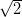–(x –) = 0
(x –)(x –) = 0x –= 0 x =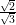x =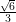….[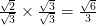Solve the equation 14/(x+3) – 1 = 5/(x+1); x ≠ -3, -1, for x. (2014D)

જવાબ :5(x + 3) = (11 – x) (x + 1)
5x + 15 = 11x + 11 – x2 – x
5x + 15 – 11x – 11 + x2 + x = 0
x2 – 5x + 4 = 0
x2 – 4x – x + 4 = 0
x(x – 4) – 1(x – 4) = 0
(x – 1) (x – 4) = 0
x – 1=0 ; x – 4 = 0
x= 1 ; x = 4

Solve the equation 3/(x+1) – ½ = 2/(3x-1) ; x ≠ -1, x ≠ 1/3 for x. (2014D)

જવાબ :1. 2(2x + 2) = (5 – x)(3x – 1)
4x + 4 = 15x – 5 – 3x2 + x
4x + 4 – 15x + 5 + 3x2 – x = 0
3x2 – 12x + 9 = 0
x2 – 4x + 3 = 0 …[Dividing the equation by 3
x2 – 3x – x + 3 = 0
x(x – 3) – 1(x – 3) = 0
(x – 1) (x – 3) = 0
x – 1 = 0 or x – 3 = 0
x = 1 ; x = 3

Solve the equation 4/x – 3 = 5/(2x+3) ≠ -1, x ≠ -3/2, for x. (2014D)

જવાબ :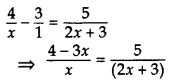5x = (2x + 3) (4 – 3x)
5x = 8x – 6x2 + 12 – 9x
5x – 8x + 6x2 – 12 + 9x = 0
6x2 + 6x – 12 = 0
x2 + x – 2 = 0 …[Dividing the equation by 6
x2 + 2x – x – 2 = 0
x(x + 2) – 1(x + 2) = 0
x – 1 = 0 or x + 2 = 0
x = 1 or x = -2

If the roots of the quadratic equation (a – b)x2 + (b – c)x + (c – a) = 0 are equal, prove that 2a = b + c. (2016OD)

જવાબ : Here ‘a’ = a – b, ‘b’ = b – c, ‘c’ = c – a
As the roots are equal

D = 0
b2 – 4ac = 0

(b – c)2 – 4(a – b)(c – a) = 0
b2 + c2 – 2bc – 4(ac – a2 – bc + ab) = 0
b2 + c2 – 2bc – 4ac + 4a2 + 4bc – 4ab = 0
4a2 + b22 + c2 – 4ab + 2bc – 4ac = 0
(-2a)2 + (b)2 + (c)22 + 2(-2a)(b) + 2(b)(c) + 2(c)(-2a) = 0
[(-2a) + (b) + (c)]2 = 0                     ….[ (x + y + z)2 = x2 + y2 + z2 + 2xy + 2yz + 2zx
Taking square-root on both sides
-2a + b + c = 0

b + c = 2a 2a = b + c

Solve for x: 3x2 – 2√23x – 23 = 0 (2015OD)

જવાબ : The given quadratic equation can be written asx2 – 2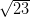x – 2= 0
a =, b = -2, c= -2D = b2 – 4ac​​​​​​​

Solve for x: 2x2 + 63x – 60 = 0 (2015OD)

જવાબ : Given equation can be written as
2(x2 + 3x – 30) = 0
x2 + 3x – 30 = 0

, a = 1, b = 3, C = -30
D = b2 – 4acFind that value of p for which the quadratic equation (p + 1)x2 – 6(p + 1)x + 3 (p + 9) = 0, p ≠ -1 had equal roots. (2015D)

જવાબ : For the given quadratic equation to have equal roots, D = 0
a = (p + 1), b = -6(p + 1), c = 3(p + 9)
D = b2 – 4ac

[-6(p + 1)]2 – 4(p + 1).3 (p + 9) = 0
36(p + 1)2 – 12(p + 1) (p + 9) = 0
12(p + 1) (3p + 3 – p – 9) = 0
12(p + 1)(2p – 6) = 0
24(p + 1)(p – 3) = 0
p + 1 = 0 or p – 3 = 0
p = -1 (rejected) or p = 3
p = 3

Find that non-zero value of k, for which the quadratic equation kx2 + 1 – 2(k – 1)x + x2 = 0 has equal roots. Hence find the roots of the equation. (2015D)

જવાબ : The given quadratic equation can be written as
kx2 + x2 – 2(k – 1)x + 1 = 0
(k + 1) x2 – 2 (k – 1) x + 1 = 0 …(A)
=>  a = (k + 1), b = -2(k – 1), c = 1
As the roots are equal, D = 0
D = b2 – 4ac

(-2(k – 1)]2 – 4 × (k + 1) × 1 = 0
4(k − 1)2 – 4(k + 1) = 0
4k2 + 4 – 8k – 4k – 4 = 0
4k2 – 12k = 0 4k(k – 3) = 0
k = 3 or k = 0 (rejected)

k = 3
Putting k = 3 put in equation (A), we get

4x2 – 4x + 1 = 0
4x2 – 2x – 2x + 1 = 0
2x(2x – 1) – 1(2x – 1) = 0
(2x – 1) (2x – 1) = 0
2x – 1 = 0 or 2x – 1 = 0
x =or x =Roots are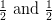For what value of k, are the roots of the quadratic equation y2 + k2 = 2 (k + 1)y equal? (2013OD)

જવાબ : y2 + k2 = 2(k + 1)y
y2 – 2(k + 1)y + k2 = 0
=> a = 1, b = -2(k + 1), c = k2
D = 0 … [Roots are equal
b2 – 4ac = 0

[-2(k + 1)]2 – 4 × (1) × (k2) = 0
4(k2 + 2k + 1) – 4k2 = 0
4k2 + 8k + 4 – 4k2 = 0
8k + 4 = 0
8k = -4 k =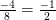For what value of k, are the roots of the quadratic equation: (k – 12)x2 + 2(k – 12)x + 2 = 0 equal? (2013OD)

જવાબ : We have, (k – 12)x2 + 2(k – 12)x + 2 = 0
The given quadratic equation will have equal roots if D = 0

b2 – 4ac = 0
=>  a = (k – 12), b = 2(k – 12), c = 2
b2 – 4ac = 0
0 = 4(k – 12)2 – 4 × (k – 12) × 2
0 = (k – 12)[4(k – 12) – 4 × 2]
0 = (k – 12) 4[k – 12 – 2]
0 = 4(k – 12) (k – 14)

4(k – 12)(k – 14) = 0
k = 12 (rejected) or k = 14
But k cannot be equal to 12 because in that case the given equation will imply 2 =
0 which is not true.
k = 14

For what values of k, the roots of the quadratic equation (k + 4)x2 + (k + 1)x + 1 = 0 are equal? (2013D)

જવાબ : We have, (k + 4) x2 + (k + 1) x + 1 = 0
=>  a = k + 4, b = k + 1, c = 1
As the roots are equal

D =0
b2 – 4ac = 0

(k + 1)2 – 4(k + 4)(1) = 0
k2 + 2k + 1 – 4k – 16 = 0
k2 – 2k – 15 = 0
k2 – 5k + 3k – 15 = 0
k(k – 5) + 3(k – 5) = 0
(k – 5)(k + 3) = 0
k – 5 = 0 or k + 3= 0
k = 5 or k = -3

k = 5 and -3

For what value of k, the roots of the quadratic equation kx(x – 25) + 10 = 0, are equal? (2013D)

જવાબ : We have, kx(x – 2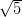) + 10 = 0
kx2 – 2kx + 10 = 0
Here a = k, b= -2k, c= 10
As the roots are equal

D = 0
As b2 – 4ac = 0
(-2k)2 – 4(k)(10) = 0
20k2 – 40k = 0

20k(k – 2) = 0
20k = 0 or k – 2 = 0
k = 0 (rejected as  Coeff. of x
2 cannot be zero) or k = 2
k= 2

Find the value of m for which the roots of the equation. mx (6x + 10) + 25 = 0, are equal. (2012OD)

જવાબ : We have, mx(6x + 10) + 25 = 0
6mx2 + 10mx + 25 = 0
Here a = 6m, b = 10m, c = 25
As the roots are equal

D = 0
b2 – 4ac = 0

(10m)2 – 4(6m) (25) = 0
100m2 – 600m = 0
100m (m – 6) = 0
100m = 0 or m – 6 = 0
m = 0 or m = 6
…[Rejecting m = 0, as coeff. of x2 cannot be zero

m = 6

Find the value of k for which the roots of the equation kx(3x – 4) + 4 = 0, are equal. (20120D)

જવાબ : We have, kx(3x – 4) + 4 = 0
3kx2 – 4kx + 4 = 0
Here a = 3k, b = -4k, c = 4
As the roots are equal

D = 0
b2 – 4ac = 0

(-4k)2 – 4(3k) (4) = 0
16k2 – 48k = 0
16k (k – 3) = 0
16k = 0 or k – 3 = 0
k = 3 or k = 0…[Rejecting k = 0, as coeff. of x2 cannot be zero

k = 3

Solve the following quadratic equation for x: x2 – 4ax – b2 + 4a2 = 0 (2012OD)

જવાબ : We have, x2 – 4ax – b2 + 4a2 = 0
x2 – 4ax + 4a2 – b2= 0
[x2 – 2(x)(2a) + (2a)2] – (b)2 = 0
(x – 2a)2 – (b)2 = 0
(x – 2a + b) (x – 2a – b) = 0
x – 2a + b = 0 or x – 2a – b = 0

x = 2a – b or x = 2a + b

Find the value(s) of k so that the quadratic equation 3x2 – 2kx + 12 = 0 has equal roots. (2012D)

જવાબ : Given: 3x2 – 2kx + 12 = 0
Here a = 3, b = -2k, c = 12
As the roots are equal

D = 0
b2 – 4ac = 0

(-2k)2 – 4(3) (12) = 0
4k2 – 144 = 0 k2 =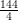= 36
k =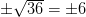Find the value(s) of k so that the quadratic equation 2x2 + kx + 3 = 0 has equal roots. (2012D)

જવાબ : Given: 2x2 + kx + 3 = 0
Here a = 2, b = k, c= 3
As the roots are equal

D = 0
As b2 – 4ac = 0
K2 – 4(2)(3) = 0
K2 – 24 = 0 or k2 = 24

k =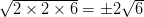### There are No Content Availble For this Chapter

Find the roots of the solution

 1    4x2−4a2x+(a4−b4)=0 A    −(a+3) and (a−2) 2    x2+5x−(a2+a−6)=0 B    a−2b and a+2b 3    x2−2ax−(4b2−a2)=0 C    (a2+b2)/2 and (a2−b2)/2 4    x2−(2b−1)x+(b2−b−20)=0 D    b−5and b+4

જવાબ :

1-C, 2-A, 3-B, 4-D

Find the roots of the solution

 1    x2−(1+√2)x+√2=0 A    ¼ 2    9x2+6x+1=0 B    √2 and 1 3    100x2−20x+1=0 C    −1/3 4    2x2−x+1/8=0 D    1/10

જવાબ :

1-B, 2-C, 3-D, 4-A

Find the roots of the solution

 1    x2−(√3+1)x+√3=0 A     −1 and −8/5 2    x2+3√3x−30=0 B    −√2 and −5√2/2 3    √2x2+7x+5√2=0 C    −5√3 and 2√3. 4    5x2+13x+8=0 D    1 and √3

જવાબ :

1-D, 2-C, 3-B, 4-A

Find the roots of the solution

 1    4√6x2−13x−2√6=0 A    √6 and √6/3 2    3x2−2√6x+2=0 B    √5 and 2√5 3    √3x2−2√2x−2√3=0 C    √6/3 4    x2−3√5x+10=0 D    −6√8 and 26√3

જવાબ :

1-D, 2-C, 3-A, 4-B

Find the roots of the solution

 1    √3x2+10x−8√3=0 A    −7√3 and 7√7 2    √3x2+11x+6√3 =0 B    −√7 and 13√7/7 3    3√7x2+4x−√7=0 C    −3√3 and −2√3/3 4    √7x2−6x−13√7=0 D    2/√3 and −4√3

જવાબ :

1-D, 2-C, 3-A, 4-B

Find the roots of the solution

 1    15x2−28=x A    −3√2 and 2√2. 2    4−11x=3x2 B    −1/16 and 1/3 3    48x2−13x−1=0 C    −4 and 1/3 4    x2+2√x−6=0 D    −4/3 and 7/5

જવાબ :

1-D, 2-C, 3-B, 4-A

Find the roots of the solution

 1    6x2 + 11x + 3 = 0 A    25/4 and −4 2    6x2+x−12=0 B    −1/3 and −3/2 3    3x2−2x−1=0 C    4/3 and −3/2 4    4x2−9x=100 D    1 and −1/3

જવાબ :

1-B, 2-C, 3-D, 4-A

Find the roots of the solution

 1. 10x – 1/x = 3 A    -a and a/2 2    2/x2−5/x+2=0 B    1/2 and −1/5 3    2x2+ax−a2=0 C    −(a+b)/2 and (a−b)/2 4    4x2+4bx−(a2−b2)= 0 D    ½ and 2

જવાબ :

1-B, 2-D, 3-A, 4-C

Find the roots of the solution

 1    x2+6x+5=0 A    −5 and −7 2    9x2-3x-2=0 B    −1 and −5 3    x2+12x+35=0 C    7 and 11 4    x2=18x−77 D    -1/3 and 2/3

જવાબ :

1-B, 2-D, 3-A, 4-C

Find the roots of the solution

 1    (2x − 3)(3x + 1) = 0 A    0 and -5/4 2    4x2 + 5x = 0 B    −9 and 9 3    3x2-243=0 C    3/2 and -1/3 4    2x2+x-6=0 D    -2 and -3/2

જવાબ :

1-C, 2-A, 3-B, 4-D

### Take a Test

Choose your Test :